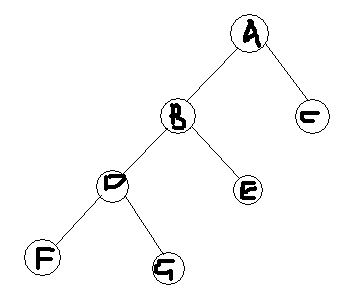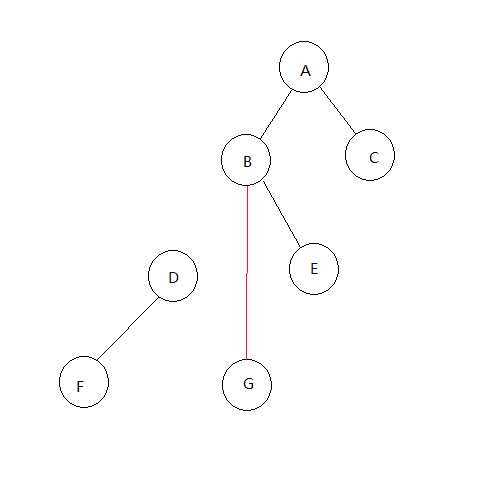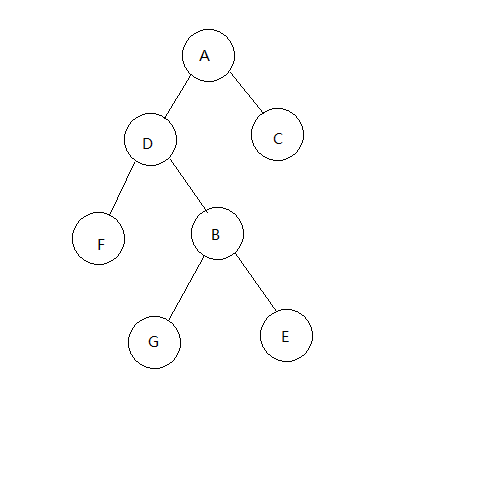# 史上最详尽的平衡树(splay)讲解与模板

【clear操作】：将当前点的各项值都清0（用于删除之后）

inline void clear(int x){
ch[x]=ch[x]=f[x]=cnt[x]=key[x]=size[x]=0;
}

【get操作】：判断当前点是它父结点的左儿子还是右儿子

inline int get(int x){
return ch[f[x]]==x;
}

【update操作】：更新当前点的size值（用于发生修改之后）

inline void update(int x){
if (x){
size[x]=cnt[x];
if (ch[x]) size[x]+=size[ch[x]];
if (ch[x]) size[x]+=size[ch[x]];
}
}

【rotate操作图文详解】step 1：

step 2：step 3：update一下当前点和各个父结点的各个值

【代码】

inline void rotate(int x){
int old=f[x],oldf=f[old],which=get(x);
ch[old][which]=ch[x][which^1];f[ch[old][which]]=old;
f[old]=x;ch[x][which^1]=old;
f[x]=oldf;
if (oldf)
ch[oldf][ch[oldf]==old]=x;
update(old);update(x);
}

【splay操作】

splay的过程中需要分类讨论，如果是三点一线的话（x，x的父亲，x的祖父）需要先rotate x的父亲，否则需要先rotate x本身（否则会形成单旋使平衡树失衡）

inline void splay(int x){
for (int fa;(fa=f[x]);rotate(x))
if (f[fa])
rotate((get(x)==get(fa)?fa:x));
root=x;
}

【insert操作】

step 1：如果root=0，即树为空的话，做一些特殊的处理，直接返回即可。

step 2：按照二叉查找树的方法一直向下找，其中：

inline void insert(int v){
if (root==0) {sz++;ch[sz]=ch[sz]=f[sz]=0;key[sz]=v;cnt[sz]=1;size[sz]=1;root=sz;return;}
int now=root,fa=0;
while (1){
if (key[now]==v){
cnt[now]++;update(now);update(fa);splay(now);break;
}
fa=now;
now=ch[now][key[now]<v];
if (now==0){
sz++;
ch[sz]=ch[sz]=0;key[sz]=v;size[sz]=1;
cnt[sz]=1;f[sz]=fa;ch[fa][key[fa]<v]=sz;
update(fa);
splay(sz);
break;
}
}
}

【find操作】查询x的排名

inline int find(int v){
int ans=0,now=root;
while (1){
if (v<key[now])
now=ch[now];
else{
ans+=(ch[now]?size[ch[now]]:0);
if (v==key[now]) {splay(now);return ans+1;}
ans+=cnt[now];
now=ch[now];
}
}
}

【findx操作】找到排名为x的点

inline int findx(int x){
int now=root;
while (1){
if (ch[now]&&x<=size[ch[now]])
now=ch[now];
else{
int temp=(ch[now]?size[ch[now]]:0)+cnt[now];
if (x<=temp)
return key[now];
x-=temp;now=ch[now];
}
}
}


【求x的前驱（后继），前驱（后继）定义为小于（大于）x，且最大（最小）的数】

【pre/next操作】

inline int pre(){
int now=ch[root];
while (ch[now]) now=ch[now];
return now;
}

inline int next(){
int now=ch[root];
while (ch[now]) now=ch[now];
return now;
}

【del操作】

step 1：随便find一下x。目的是：将x旋转到根。

step 2：那么现在x就是根了。如果cnt[root]>1，即不只有一个x的话，直接-1返回。

step 3：如果root并没有孩子，就说名树上只有一个x而已，直接clear返回。

step 4：如果root只有左儿子或者右儿子，那么直接clear root，然后把唯一的儿子当作根就可以了（f赋0，root赋为唯一的儿子）

step 5：我们找到新根，也就是x的前驱（x左子树最大的一个点），将它旋转到根。然后将原来x的右子树接到新根的右子树上（注意这个操作需要改变父子关系）。这实际上就把x删除了。不要忘了update新根。

inline void del(int x){
int whatever=find(x);
if (cnt[root]>1) {cnt[root]--;update(root);return;}
//Only One Point
if (!ch[root]&&!ch[root]) {clear(root);root=0;return;}
//Only One Child
if (!ch[root]){
int oldroot=root;root=ch[root];f[root]=0;clear(oldroot);return;
}
else if (!ch[root]){
int oldroot=root;root=ch[root];f[root]=0;clear(oldroot);return;
}
//Two Children
int leftbig=pre(),oldroot=root;
splay(leftbig);
f[ch[oldroot]]=root;
ch[root]=ch[oldroot];
clear(oldroot);
update(root);
return;
}

【总结】

splay的本质是rotate，旋转其实只是为了保证二叉搜索树的平衡性。©️2019 CSDN 皮肤主题: 编程工作室 设计师: CSDN官方博客